• Buckinghamshire Tutors

# Buckinghamshire Grammars 11+ Course, Week 6: Verbal Reasoning, Maths and Vocabulary.Welcome to week 6 of my blog. (We have had a break while the Tutor Doctor website had an overhaul.) We will be looking at Finding the Hidden Word for Verbal Reasoning, and Sequences including, Square Numbers, Triangular numbers, Square Numbers, and of course, Primes, as well as the nth term in Maths.

This Blog will look better if you open it in Chrome

## Find the hidden word

Where you find a word hidden at the end of one word and the beginning of the next word.

Here's an example:

Do not end a good thing.

The hidden word is note.

Here's another example:
I arrived with empty pockets and left with full ones!

The hidden word is them.

Tip: Try not to read the actual sentence, but to spot the word in-between two words. Do this with every space between words until you find the hidden word.

He was hinting he wanted a new bike.

The hidden word is shin.

The hidden word may 'jump out at you,' but if not, be systematic:

- - - / -  not/e

- - / - -  th/em

- / - - -  s/hin

S and h combine to make sh as with s/hin. Similary, c and h combine to make ch,

c and e combine to make a 'soft' ce like in mice, and g and e combine to make a 'soft' ge like in fudge.

Some words end in double letters e.g. class

Pronouns are easily overlooked: (they, them, their, your, mine)

Remember, all words need a vowel, so a row of consonants at the end of one word and the beginning of another is not hopeful.

## Find the Hidden word exercises:

1. The envelope stuck in the letterbox.
2. When will you be arriving?
3. Sit over by the window.
4. The picnic ended when it rained.
5. You reek of leeks!
6. The percentage didn’t add up to 100.
7. The new hen got a new bowl.
8. The alternative was not an option.
9. The celeriac hindered digestion.
10. We shop even when the shops are shut.
11. In time all of you will learn this.
12.  The cat wasn’t his property.
13. Have you met all her family?
14. The car engine hummed.
15. His story wasn’t plausible.
16. Who met the Dr?
17. He drew early in the morning.
18. North is north.
19. The chick needs a rest.
20. The elf indulged in a cake.

Answers to Find the Hidden Word

## Types of sequences:Tip: Always write out the sequence like the example above as it helps you to see it, especially with trickier sequences.What are the next two numbers in the sequence?Practice using the game below: (start on hard)

http://www.free-training-tutorial.com/pattern/sealife/sl-pattern.htmlhttps://mathsframe.co.uk/en/resources/playgame/42http://www.free-training-tutorial.com/math-games/pattern-crack-the-safe-game.html

But what if there is no obvious sequence?

Look at these numbers: 4, 9, 25, 36, 49.

They don’t make any sense unless you know your ... square numbers because they make the shape of a square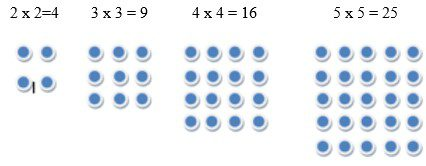Can you see the rest of the sequence?

6 x 6, 7x7, 8 x 8, 9 x 9, 10 x 10?

Ballpit: Make your own square numbers.

Triangular numbers make the shape of a triangleCan you see the sequence?

See how the number added on goes up by one more in each triangular number? 1 + 2 = 3, 3 + 3 = 6, 6 = 4 = 10, 10 + 5 =15. So the next new triangular number will have 6 added onto the previous shape.

The next triangular numbers in the sequence is: 15 + 6 = 21, then 21 + 7 = 28,  28 +8 = 36, 36 + 9 = 45, 45 + 10 = 55 and so on. They grow cumulatively (which means adding more to what was already there, again, and again, like a snowball cumulatively getting larger).

Make you own triangular numbers using the word documents below. Go up to 55.

Ballpit: Make your own triangular numbers.

Cube Numbers:https://www.bbc.co.uk/education/guides/zdkwxnb/revision/3

The Famous Fiobannaci Sequence: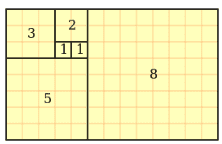The Fiobannaci sequence begins 0, 1, 1, 2, 3, 5, 8, 13, 21, 34, 55, 89, 144, 233, 377, 610, 987. Each number is the sum of the two previous numbers.

Some natural spirals follow this mathematical sequence including the Nautilus shell.Remember your primes? Which of the following numbers are primes?

TIP: two is the only even prime, 5 is the only prime that ends in a 5. 51 is not a prime, as 17 x 3=51, and 81 is not a prime e because 27 x 3= 81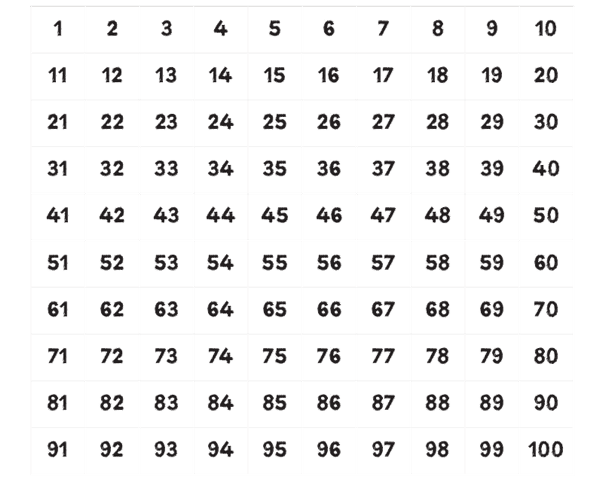## More SequencesSome sequences use division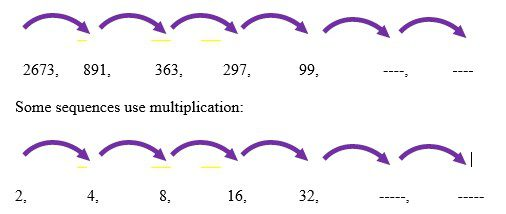## Sequences: find the missing numbers

1. 19, 28, 37, 46,   --, --
2. 67, 56, 45, 34,  --, --
3. 100, 3, 50, 7, --, --
4. 10, ---, 1000, 10000, ------
5. 28, 9, 29, 13, 30, --, --
6. 16, --, 20, --, 24
7. 72, --, --, 54, 48
8. 89, 66, 87, 69, --, --
9. --, 12, 48, 192, --
10. 41, 43, 47, --, 53
11. 8, 13, 21, 34, --, --
12. 3, 7, 10, 17, --, --
13. 125, 100, 75, --, --
14. 1.5, 3, 4.5, 6, --, --
15. 3.5, 3.6, 3.7, -.-, -.- 4.0
16. 4.25, 4.50, 4,75, -.-, 5.25
17. 47, 53, 59, ..
18. 1, 4, --, 16, 25
19. 729, 243, 81, --, --
20. 10, 7.5, 5, 2.5, --, --

Sometimes sequences follow the times tables:And some follow the times tables – sort of:The second sequence is out by 2 each time. But, that doesn’t mean we can’t use it; because we can. We can use the five times tables to predict the next numbers,so long as we remember to add on that extra two at the beginning. (We only need to add it one time at the beginning.)

We call this the nth term.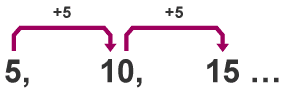Because we are using the 5 times tables, we call the above sequence 5n.Because this uses the 5 times tables, but is always out by 2, this sequence is called 5n +2.

Look at the first number, 7. We can call this the ‘first term’. Because it is the first term, n = 1, so we get 5 x 1. Don’t forget to add the 2 on.  We get 7.

Look at the second number, 12. We call this the ‘second term’. Because it is the second term n =2, so we get 5 x 2. The two has to be added on. We get 12.

Look at the third number, 17. We call this the ‘third term’. Because it is the third term n = 3, so we get 5 x 3. The two has to be added on. We get 17.

What will the sixth term be?

What will the tenth term be?

What will the fiftieth term be?

What is the nth term in the sequence 8, 11, 14?

The difference between each number is always 3, so we can use the three times tables for this.

But look at the first number, 8. We can call this the ‘first term’. The first term (8) is 5 more than three, so that 5 will be added on, all the way through the sequence. And the nth term is 3n + 5.

3n+5 is the first term, and because it is the first term we multiply the 3 by 1 (and add 5) so we get the number 8

3n+5 is the second term, but because it is the second term we multiply the 3 by 2 (and add 5) so we get the number 11

3n + 5 is the third term, but because it is the third term we multiply the 3 by 3 (and add 5) so we get the number 14

What is the fourth term?

What is the fifth term?

What is the ninth term

Here’s a clip.https://www.bbc.co.uk/education/guides/z7j2pv4/video

Now do the self-marking test (below).

This weeks words:

Learn the meaning of each word, the synonyms, the antonyms, draw a stick person picture that will help you remember: put each word and one antonym into seperate sentences.Next Week

Fractions

Verbal Reasoning

non-Verbal Reasoning